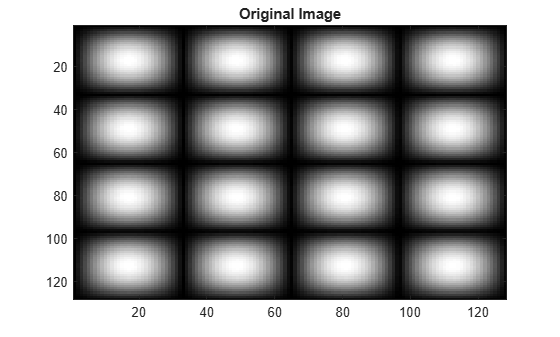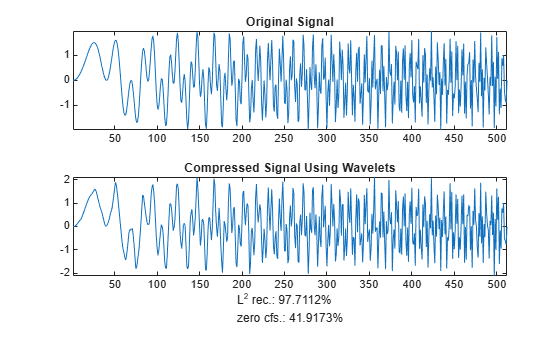# wpdencmp

Denoising or compression using wavelet packets

## Syntax

``[xd,treed,perf0,perfl2] = wpdencmp(x,sorh,n,wname,crit,par,keepapp)``
``[___] = wpdencmp(tree,sorh,crit,par,keepapp)``

## Description

`wpdencmp` performs a denoising or compression process of a signal or image using wavelet packets. The ideas and procedures for denoising and compression using either wavelet or wavelet packet decompositions are the same. See `wdenoise` or `wdencmp` for more information.

example

````[xd,treed,perf0,perfl2] = wpdencmp(x,sorh,n,wname,crit,par,keepapp)` returns a denoised or compressed version `xd` of the input data `x` obtained by wavelet packet coefficient thresholding. `wpdencmp` also returns the wavelet packet best tree decomposition `treed` of `xd` (see `besttree` for more information), and the L2 energy recovery and compression scores in percentages as `perfl2` and `perf0`, respectively.```
````[___] = wpdencmp(tree,sorh,crit,par,keepapp)` uses the wavelet packet decomposition `tree` of the data to be denoised or compressed.```

## Examples

collapse all

This example shows how to denoise using wavelet packets.

Use `wnoise` to generate the heavy sine signal and a noisy version.

```init = 1000; [xref,x] = wnoise(5,11,7,init); figure subplot(2,1,1) plot(xref) axis tight title('Heavy Sine') subplot(2,1,2) plot(x) axis tight title('Noisy Heavy Sine')```Denoise the noisy signal using a four-level wavelet packet decomposition. Use the order 4 Daubechies least asymmetric wavelet.

```n = length(x); thr = sqrt(2*log(n*log(n)/log(2))); xwpd = wpdencmp(x,'s',4,'sym4','sure',thr,1);```

Compare with a wavelet-based denoising result. Use `wdenoise` with comparable input arguments. Plot the differences between the two denoised signals and original signal.

```xwd = wdenoise(x,4,'Wavelet','sym4','DenoisingMethod','UniversalThreshold','ThresholdRule','Hard'); figure subplot(2,1,1) plot(x-xwpd) axis tight ylim([-12 12]) title('Difference Between Wavelet Packet Denoised and Original') subplot(2,1,2) plot(x-xwd) axis tight ylim([-12 12]) title('Difference Between Wavelet Denoised and Original')```This example shows how to denoise an image using wavelet packets.

Load an image and generate a noisy copy. For reproducibility set the random seed.

```rng default load sinsin x = X/18 + randn(size(X)); imagesc(X) colormap(gray) title('Original Image')``````figure imagesc(x) colormap(gray) title('Noisy Image')```Denoise the noisy image using wavelet packet decomposition. Use `ddencmp` to determine denoising parameters. Do a three-level decomposition with the order 4 Daubechies least asymmetric wavelet.

```[thr,sorh,keepapp,crit] = ddencmp('den','wp',x); xd = wpdencmp(x,sorh,3,'sym4',crit,thr,keepapp); figure imagesc(xd) colormap(gray) title('Denoised Image')```This example shows how to compress a 1-D signal using wavelet packets.

Load a signal. Use `ddencmp` to determine compression values for that signal.

```load sumlichr x = sumlichr; [thr,sorh,keepapp,crit] = ddencmp('cmp','wp',x)```
```thr = 0.5193 ```
```sorh = 'h' ```
```keepapp = 1 ```
```crit = 'threshold' ```

Compress the signal using global thresholding with threshold best basis. Use the order 4 Daubechies least asymmetric wavelet and do a three-level wavelet packet decomposition.

`[xc,wpt,perf0,perfl2] = wpdencmp(x,sorh,3,'sym4',crit,thr,keepapp);`

Compare the original signal with the compressed version.

```subplot(2,1,1) plot(x) title('Original Signal') axis tight subplot(2,1,2) plot(xc) xlabel(['L^2 rec.: ',num2str(perfl2),'% zero cfs.: ',num2str(perf0),'%']) title('Compressed Signal Using Wavelet Packets') axis tight```Compress the signal again, but this do a three-level wavelet decomposition. Keep all the other parameters the same.

```[thr,sorh,keepapp] = ddencmp('cmp','wv',x); [xcwv,~,~,perf0wv,perfl2wv] = wdencmp('gbl',x,'sym4',3,thr,sorh,keepapp); figure subplot(2,1,1) plot(x) title('Original Signal') axis tight subplot(2,1,2) plot(xc) xlabel(['L^2 rec.: ',num2str(perfl2wv),'% zero cfs.: ',num2str(perf0wv),'%']) title('Compressed Signal Using Wavelets') axis tight```A larger fraction of coefficients are set equal to 0 when compressing using a wavelet packet decomposition.

## Input Arguments

collapse all

Input data to denoise or compress, specified by a real-valued vector or matrix.

Data Types: `double`

Wavelet packet decomposition of the data to be denoised or compressed, specified as a wavelet packet tree. See `wpdec` and `wpdec2` for more information.

Type of thresholding to perform:

• `'s'` — Soft thresholding

• `'h'` — Hard thresholding

See `wthresh` for more information.

Wavelet packet decomposition level, specified as a positive integer.

Name of wavelet, specified as a character vector or string scalar, to use for denoising. See `wavemngr` for more information.

Entropy type, specified as one of the following:

Entropy Type (`crit`)

Threshold Parameter (`par`)

`'shannon'`

`par` is not used.

`'log energy'`

`par` is not used.

`'threshold'``0 ≤ par`

`par` is the threshold.

`'sure'``0 ≤ par`

`par` is the threshold.

`'norm'``1 ≤ par`

`par` is the power.

`'user'`Character vector

`par` is a character vector containing the file name of your own entropy function, with a single input `x`.

'`FunName`'No constraints on `par`

`FunName` is any character vector other than the previous entropy types listed.

`FunName` contains the file name of your own entropy function, with `x` as input and `par` as an additional parameter to your entropy function.

`crit` and threshold parameter `par` together define the entropy criterion used to determine the best decomposition. See `wentropy` for more information.

If `crit = 'nobest'`, no optimization is done, and the current decomposition is thresholded.

Threshold parameter, specified by a real number, character vector, or string scalar. `par` and the entropy type `crit` together define the entropy criterion used to determine the best decomposition. See `wentropy` for more information.

Data Types: `double`

Threshold approximation setting, specified as either `0` or `1`. If `keepapp = 1`, the approximation coefficients cannot be thresholded. If ```keepapp = 0```, the approximation coefficients can be thresholded.

Data Types: `double`

## Output Arguments

collapse all

Denoised or compressed data, returned as a real-valued vector or matrix. `xd` and `x` have the same dimensions.

Wavelet packet best tree decomposition of `xd`, returned as a wavelet packet tree.

Compression score, returned as a real number. `perf0` is the percentage of thresholded coefficients that are equal to 0.

L2 energy recovery, returned as a real number. `perfl2` is equal to If `x` is a one-dimensional signal and `wname` an orthogonal wavelet, `perfl2` simplifies to $\frac{100{‖xd‖}^{2}}{{‖x‖}^{2}}.$

 Antoniadis, A., and G. Oppenheim, eds. Wavelets and Statistics. Lecture Notes in Statistics. New York: Springer Verlag, 1995.

 Coifman, R. R., and M. V. Wickerhauser. “Entropy-Based Algorithms for Best Basis Selection.” IEEE Transactions on Information Theory. Vol. 38, Number 2, 1992, pp. 713–718.

 DeVore, R. A., B. Jawerth, and B. J. Lucier. “Image Compression Through Wavelet Transform Coding.” IEEE Transactions on Information Theory. Vol. 38, Number 2, 1992, pp. 719–746.

 Donoho, D. L. “Progress in Wavelet Analysis and WVD: A Ten Minute Tour.” Progress in Wavelet Analysis and Applications (Y. Meyer, and S. Roques, eds.). Gif-sur-Yvette: Editions Frontières, 1993.

 Donoho, D. L., and I. M. Johnstone. “Ideal Spatial Adaptation by Wavelet Shrinkage.” Biometrika. Vol. 81, 1994, pp. 425–455.

 Donoho, D. L., I. M. Johnstone, G. Kerkyacharian, and D. Picard. “Wavelet Shrinkage: Asymptopia?” Journal of the Royal Statistical Society, series B. Vol. 57, Number 2, 1995, pp. 301–369.